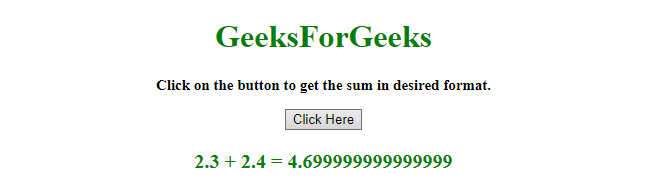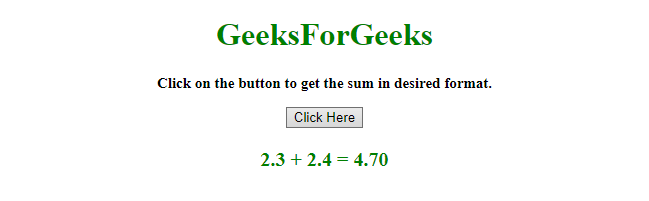# How to add float numbers using JavaScript ?

Given two or more numbers and the task is to get the float addition in the desired format with the help of JavaScript. There are two methods to solve this problem which are discussed below:

Approach 1:

Example: This example implements the above approach.

 `  ` `<``html``>  ` ` `  `<``head``>  ` `    ``<``title``>  ` `        ``Float sum with JavaScript. ` `    `` ` `  ` ` `  `<``body` `style` `= ``"text-align:center;"``>  ` `     `  `    ``<``h1` `style` `= ``"color:green;"` `>  ` `        ``GeeksForGeeks  ` `    `` ` `     `  `    ``<``p` `id` `= ``"GFG_UP"` `style` `= ` `        ``"font-size: 15px; font-weight: bold;"``> ` `    `` ` `     `  `    ``<``button` `onclick` `= ``"gfg_Run()"``>  ` `        ``Click Here ` `    `` ` `     `  `    ``<``p` `id` `= ``"GFG_DOWN"` `style = "color:green; ` `        ``font-size: 20px; font-weight: bold;"> ` `    `` ` `     `  `    ``<``script``> ` `        ``var el_up = document.getElementById("GFG_UP"); ` `        ``var el_down = document.getElementById("GFG_DOWN"); ` `         `  `        ``el_up.innerHTML = "Click on the button to get the" ` `                          ``+ " sum in desired format."; ` `         `  `        ``var val = parseFloat('2.3')+parseFloat('2.4'); ` `        ``el_down.innerHTML = "2.3 + 2.4 = " + val; ` `         `  `        ``function gfg_Run() { ` `            ``el_down.innerHTML = "2.3 + 2.4 = "  ` `                        ``+ (parseFloat('2.3') +  ` `                        ``parseFloat('2.4')).toFixed(2); ` `        ``}  ` `    ``  ` `  ` ` `  ` `

Output:

• Before clicking on the button:• After clicking on the button:Approach 2:

Example: This example implements the above approach.

 `  ` `<``html``>  ` ` `  `<``head``>  ` `    ``<``title``>  ` `        ``Float sum with javascript. ` `    `` ` `  ` ` `  `<``body` `style` `= ``"text-align:center;"``>  ` `     `  `    ``<``h1` `style` `= ``"color:green;"` `>  ` `        ``GeeksForGeeks  ` `    `` ` `     `  `    ``<``p` `id` `= ``"GFG_UP"` `style` `= ` `        ``"font-size: 15px; font-weight: bold;"``> ` `    `` ` `     `  `    ``<``button` `onclick` `= ``"gfg_Run()"``>  ` `        ``Click Here ` `    `` ` `     `  `    ``<``p` `id` `= ``"GFG_DOWN"` `style = "color:green; ` `        ``font-size: 20px; font-weight: bold;"> ` `    `` ` `     `  `    ``<``script``> ` `        ``var el_up = document.getElementById("GFG_UP"); ` `        ``var el_down = document.getElementById("GFG_DOWN"); ` `         `  `        ``el_up.innerHTML = "Click on the button to get the" ` `                          ``+ " sum in desired format."; ` `         `  `        ``var val = parseFloat('2.3')+parseFloat('2.4'); ` `        ``el_down.innerHTML = "2.3 + 2.4 = " + val; ` `         `  `        ``function gfg_Run() { ` `            ``el_down.innerHTML = "2.3 + 2.4 = " +  ` `                    ``Math.round((parseFloat('2.3') ` `                    ``+ parseFloat('2.4'))*100)/100; ` `        ``}  ` `    ``  ` `  ` ` `  ` `

Output:

• Before clicking on the button:• After clicking on the button:My Personal Notes arrow_drop_upCheck out this Author's contributed articles.

If you like GeeksforGeeks and would like to contribute, you can also write an article using contribute.geeksforgeeks.org or mail your article to contribute@geeksforgeeks.org. See your article appearing on the GeeksforGeeks main page and help other Geeks.

Please Improve this article if you find anything incorrect by clicking on the "Improve Article" button below.